# Creating custom plots

While ArviZ includes many plotting functions for visualizing the data stored in InferenceData objects, you will often need to construct custom plots, or you may want to tweak some of our plots in your favorite plotting package.

In this tutorial, we will show you a few useful techniques you can use to construct these plots using Julia's plotting packages. For demonstration purposes, we'll use Makie.jl and AlgebraOfGraphics.jl, which can consume Dataset objects since they implement the Tables interface. However, we could just as easily have used StatsPlots.jl.

begin
using ArviZ, DimensionalData, DataFrames, Statistics, AlgebraOfGraphics, CairoMakie
using AlgebraOfGraphics: density
set_aog_theme!()
end;

We'll start by loading some draws from an implementation of the non-centered parameterization of the 8 schools model. In this parameterization, the model has some sampling issues.

idata = load_example_data("centered_eight")
InferenceData
posterior
Dataset with dimensions:
Dim{:chain} Sampled 0:3 ForwardOrdered Regular Points,
Dim{:draw} Sampled 0:499 ForwardOrdered Regular Points,
Dim{:school} Categorical String[Choate, Deerfield, …, St. Paul's, Mt. Hermon] Unordered
and 3 layers:
:mu    Float64 dims: Dim{:chain}, Dim{:draw} (4×500)
:theta Float64 dims: Dim{:chain}, Dim{:draw}, Dim{:school} (4×500×8)
:tau   Float64 dims: Dim{:chain}, Dim{:draw} (4×500)

with metadata OrderedCollections.OrderedDict{Symbol, Any} with 3 entries:
:created_at => "2019-06-21T17:36:34.398087"
:inference_library_version => "3.7"
:inference_library => "pymc3"
posterior_predictive
Dataset with dimensions:
Dim{:chain} Sampled 0:3 ForwardOrdered Regular Points,
Dim{:draw} Sampled 0:499 ForwardOrdered Regular Points,
Dim{:school} Categorical String[Choate, Deerfield, …, St. Paul's, Mt. Hermon] Unordered
and 1 layer:
:obs Float64 dims: Dim{:chain}, Dim{:draw}, Dim{:school} (4×500×8)

with metadata OrderedCollections.OrderedDict{Symbol, Any} with 3 entries:
:created_at => "2019-06-21T17:36:34.489022"
:inference_library_version => "3.7"
:inference_library => "pymc3"
sample_stats
Dataset with dimensions:
Dim{:chain} Sampled 0:3 ForwardOrdered Regular Points,
Dim{:draw} Sampled 0:499 ForwardOrdered Regular Points,
Dim{:school} Categorical String[Choate, Deerfield, …, St. Paul's, Mt. Hermon] Unordered
and 12 layers:
:tune             Bool dims: Dim{:chain}, Dim{:draw} (4×500)
:depth            Int64 dims: Dim{:chain}, Dim{:draw} (4×500)
:tree_size        Float64 dims: Dim{:chain}, Dim{:draw} (4×500)
:lp               Float64 dims: Dim{:chain}, Dim{:draw} (4×500)
:energy_error     Float64 dims: Dim{:chain}, Dim{:draw} (4×500)
:step_size_bar    Float64 dims: Dim{:chain}, Dim{:draw} (4×500)
:max_energy_error Float64 dims: Dim{:chain}, Dim{:draw} (4×500)
:energy           Float64 dims: Dim{:chain}, Dim{:draw} (4×500)
:mean_tree_accept Float64 dims: Dim{:chain}, Dim{:draw} (4×500)
:step_size        Float64 dims: Dim{:chain}, Dim{:draw} (4×500)
:diverging        Bool dims: Dim{:chain}, Dim{:draw} (4×500)
:log_likelihood   Float64 dims: Dim{:chain}, Dim{:draw}, Dim{:school} (4×500×8)

with metadata OrderedCollections.OrderedDict{Symbol, Any} with 3 entries:
:created_at => "2019-06-21T17:36:34.485802"
:inference_library_version => "3.7"
:inference_library => "pymc3"
prior
Dataset with dimensions:
Dim{:chain} Sampled StepRangeLen(0.0, 0.0, 1) ForwardOrdered Regular Points,
Dim{:draw} Sampled 0:499 ForwardOrdered Regular Points,
Dim{:school} Categorical String[Choate, Deerfield, …, St. Paul's, Mt. Hermon] Unordered
and 5 layers:
:tau       Float64 dims: Dim{:chain}, Dim{:draw} (1×500)
:tau_log__ Float64 dims: Dim{:chain}, Dim{:draw} (1×500)
:mu        Float64 dims: Dim{:chain}, Dim{:draw} (1×500)
:theta     Float64 dims: Dim{:chain}, Dim{:draw}, Dim{:school} (1×500×8)
:obs       Float64 dims: Dim{:chain}, Dim{:draw}, Dim{:school} (1×500×8)

with metadata OrderedCollections.OrderedDict{Symbol, Any} with 3 entries:
:created_at => "2019-06-21T17:36:34.490387"
:inference_library_version => "3.7"
:inference_library => "pymc3"
observed_data
Dataset with dimensions:
Dim{:school} Categorical String[Choate, Deerfield, …, St. Paul's, Mt. Hermon] Unordered
and 1 layer:
:obs Float64 dims: Dim{:school} (8)

with metadata OrderedCollections.OrderedDict{Symbol, Any} with 3 entries:
:created_at => "2019-06-21T17:36:34.491909"
:inference_library_version => "3.7"
:inference_library => "pymc3"
idata.posterior
Dataset with dimensions:
Dim{:chain} Sampled 0:3 ForwardOrdered Regular Points,
Dim{:draw} Sampled 0:499 ForwardOrdered Regular Points,
Dim{:school} Categorical String[Choate, Deerfield, …, St. Paul's, Mt. Hermon] Unordered
and 3 layers:
:mu    Float64 dims: Dim{:chain}, Dim{:draw} (4×500)
:theta Float64 dims: Dim{:chain}, Dim{:draw}, Dim{:school} (4×500×8)
:tau   Float64 dims: Dim{:chain}, Dim{:draw} (4×500)

with metadata OrderedCollections.OrderedDict{Symbol, Any} with 3 entries:
:created_at                => "2019-06-21T17:36:34.398087"
:inference_library_version => "3.7"
:inference_library         => "pymc3"

The plotting functions we'll be using interact with a tabular view of a Dataset. Let's see what that view looks like for a Dataset:

df = DataFrame(idata.posterior)
chain draw school mu theta tau
1 0 0 "Choate" -3.47699 1.66865 3.7301
2 1 0 "Choate" 8.25086 8.09621 1.19333
3 2 0 "Choate" 10.5171 14.5709 5.13725
4 3 0 "Choate" 4.5323 4.32639 0.50007
5 0 1 "Choate" -2.45587 -6.23936 2.07538
6 1 1 "Choate" 8.25086 8.09621 1.19333
7 2 1 "Choate" 9.88795 12.6867 4.26438
8 3 1 "Choate" 4.5323 4.32639 0.50007
9 0 2 "Choate" -2.82625 2.1951 3.70299
10 1 2 "Choate" 8.25086 8.09621 1.19333
...
16000 3 499 "Mt. Hermon" 0.161389 4.52339 5.4068

The tabular view includes dimensions and variables as columns.

When variables with different dimensions are flattened into a tabular form, there's always some duplication of values. As a simple case, note that chain, draw, and school all have repeated values in the above table.

In this case, theta has the school dimension, but tau doesn't, so the values of tau will be repeated in the table for each value of school.

df[df.school .== Ref("Choate"), :].tau == df[df.school .== Ref("Deerfield"), :].tau
true

In our first example, this will be important.

Here, let's construct a trace plot. Besides idata, all functions and types in the following cell are defined in AlgebraOfGraphics or Makie:

• data(...) indicates that the wrapped object implements the Tables interface

• mapping indicates how the data should be used. The symbols are all column names in the table, which for us are our variable names and dimensions.

• visual specifies how the data should be converted to a plot.

• Lines is a plot type defined in Makie.

• draw takes this combination and plots it.

draw(
data(idata.posterior.mu) *
mapping(:draw, :mu; color=:chain => nonnumeric) *
visual(Lines; alpha=0.8),
)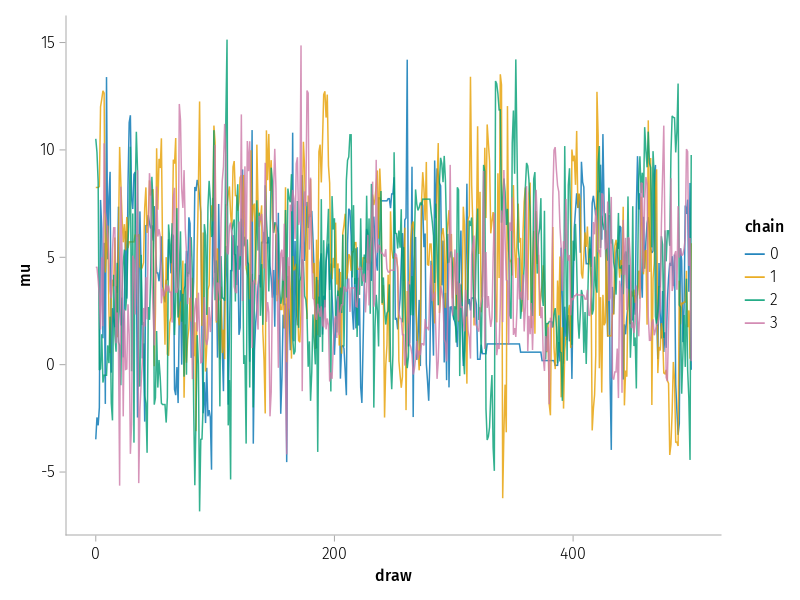Note the line idata.posterior.mu. If we had just used idata.posterior, the plot would have looked more-or-less the same, but there would be artifacts due to mu being copied many times. By selecting mu directly, all other dimensions are discarded, so each value of mu appears in the plot exactly once.

When examining an MCMC trace plot, we want to see a "fuzzy caterpillar". Instead we see a few places where the Markov chains froze. We can do the same for theta as well, but it's more useful here to separate these draws by school.

draw(
data(idata.posterior) *
mapping(:draw, :theta; layout=:school, color=:chain => nonnumeric) *
visual(Lines; alpha=0.8),
)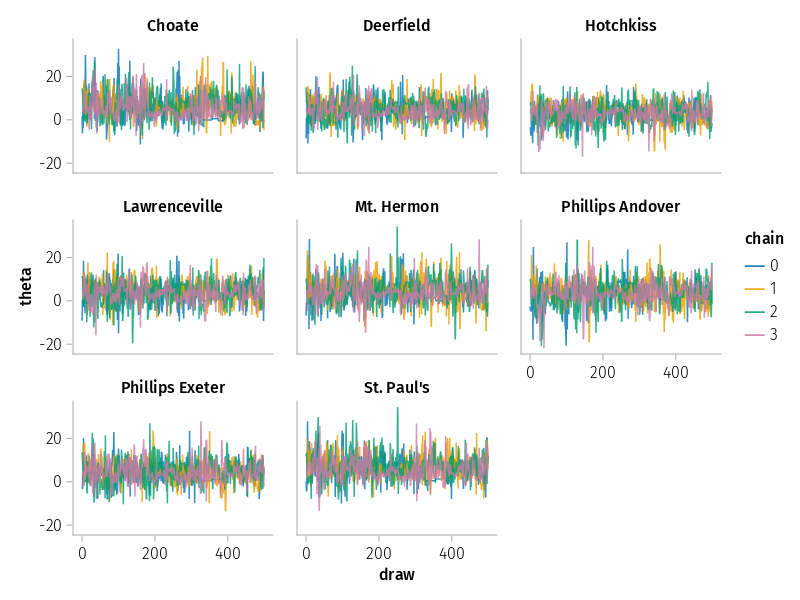Suppose we want to compare tau with theta for two different schools. To do so, we use InferenceDatas indexing syntax to subset the data.

draw(
data(idata[:posterior, school=At(["Choate", "Deerfield"])]) *
mapping(:theta, :tau; color=:school) *
density() *
visual(Contour; levels=10),
)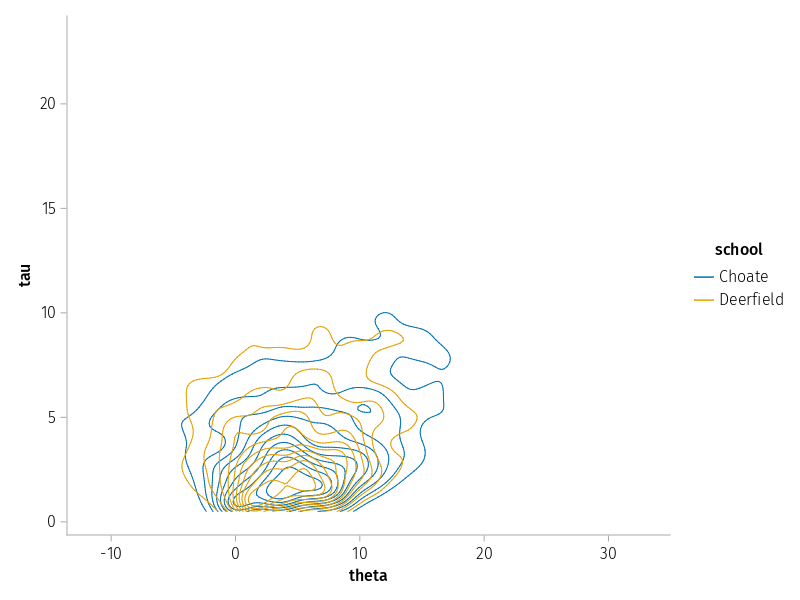We can also compare the density plots constructed from each chain for different schools.

draw(
data(idata.posterior) *
mapping(:theta; layout=:school, color=:chain => nonnumeric) *
density(),
)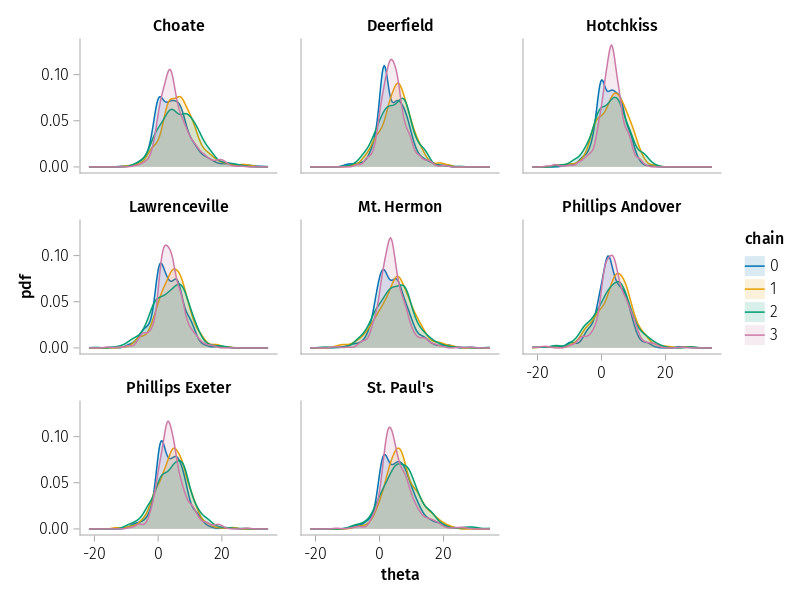If we want to compare many schools in a single plot, an ECDF plot is more convenient.

draw(
data(idata.posterior) * mapping(:theta; color=:school => nonnumeric) * visual(ECDFPlot);
axis=(; ylabel="probability"),
)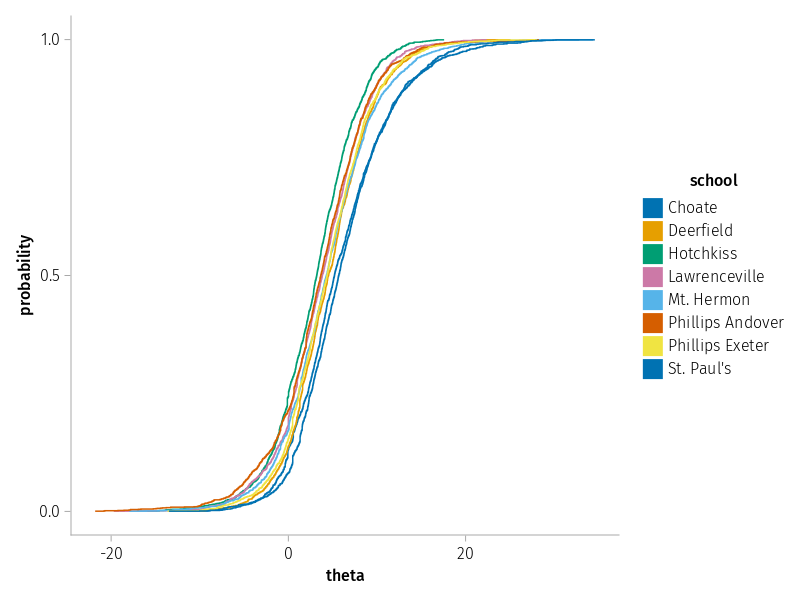So far we've just plotted data from one group, but we often want to combine data from multiple groups in one plot. The simplest way to do this is to create the plot out of multiple layers. Here we use this approach to plot the observations over the posterior predictive distribution.

draw(
(data(idata.posterior_predictive) * mapping(:obs; layout=:school) * density()) +
(data(idata.observed_data) * mapping(:obs, :obs => zero => ""; layout=:school)),
)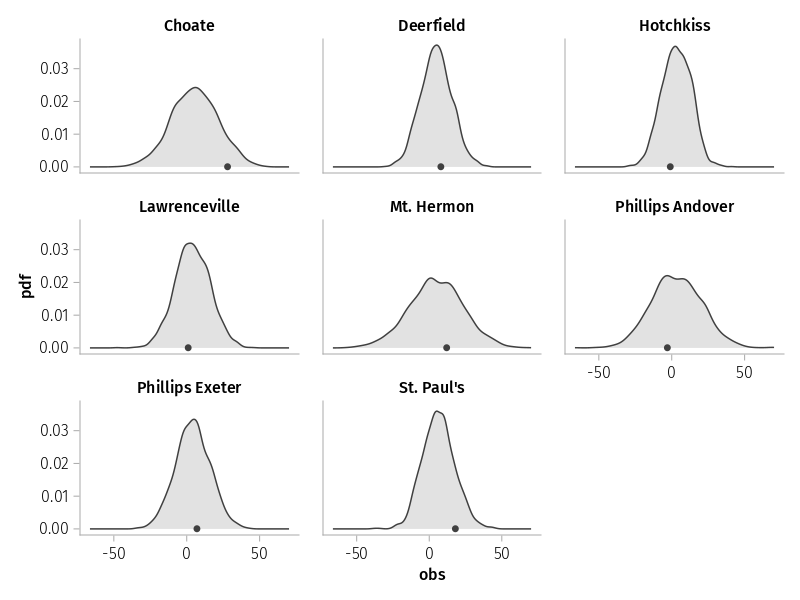Another option is to combine the groups into a single dataset.

Here we compare the prior and posterior. Since the prior has 1 chain and the posterior has 4 chains, if we were to combine them into a table, the structure would need to be ragged. This is not currently supported.

We can then either plot the two distributions separately as we did before, or we can compare a single chain from each group. This is what we'll do here. To concatenate the two groups, we introduce a new named dimension using DimensionalData.Dim.

draw(
data(
cat(
idata.posterior[chain=], idata.prior; dims=Dim{:group}([:posterior, :prior])
)[:mu],
) *
mapping(:mu; color=:group) *
histogram(; bins=20) *
visual(; alpha=0.8);
axis=(; ylabel="probability"),
)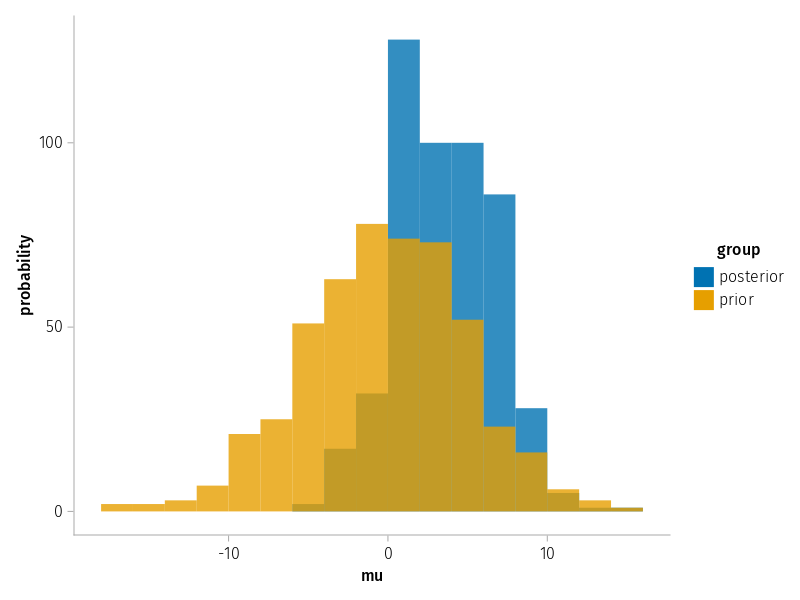From the trace plots, we suspected the geometry of this posterior was bad. Let's highlight divergent transitions. To do so, we merge posterior and samplestats, which can do with merge since they share no common variable names.

draw(
data(merge(idata.posterior, idata.sample_stats)) * mapping(
:theta,
:tau;
layout=:school,
color=:diverging,
markersize=:diverging => (d -> d ? 5 : 2),
),
)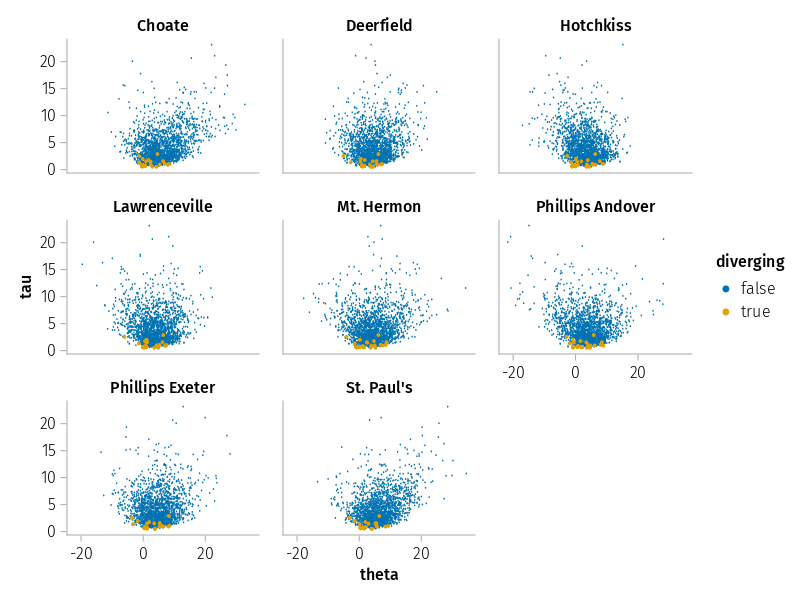When we try building more complex plots, we may need to build new Datasets from our existing ones.

One example of this is the corner plot. To build this plot, we need to make a copy of theta with a copy of the school dimension.

let
theta = idata.posterior.theta[school=1:4]
theta2 = rebuild(set(theta; school=:school2); name=:theta2)
plot_data = Dataset(theta, theta2, idata.sample_stats.diverging)
draw(
data(plot_data) * mapping(
:theta,
:theta2 => "theta";
col=:school,
row=:school2,
color=:diverging,
markersize=:diverging => (d -> d ? 3 : 1),
);
figure=(; figsize=(5, 5)),
axis=(; aspect=1),
)
end